Скачать презентацию 2011 Cengage Learning California Real Estate

• Количество слайдов: 24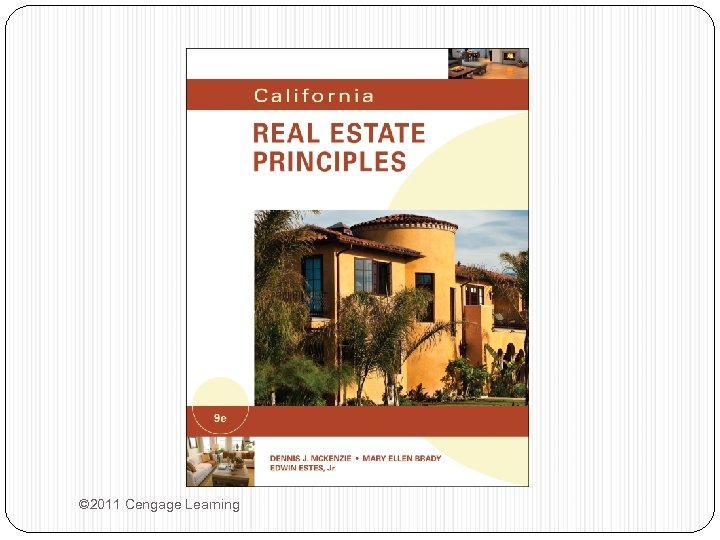© 2011 Cengage Learning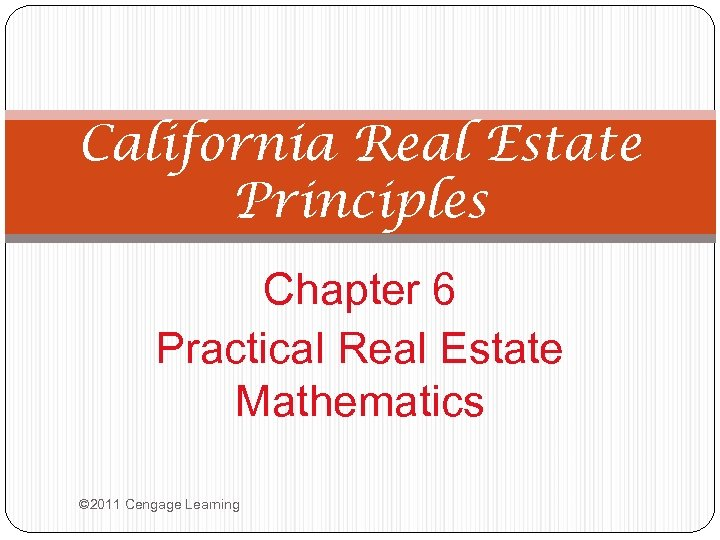California Real Estate Principles Chapter 6 Practical Real Estate Mathematics © 2011 Cengage Learning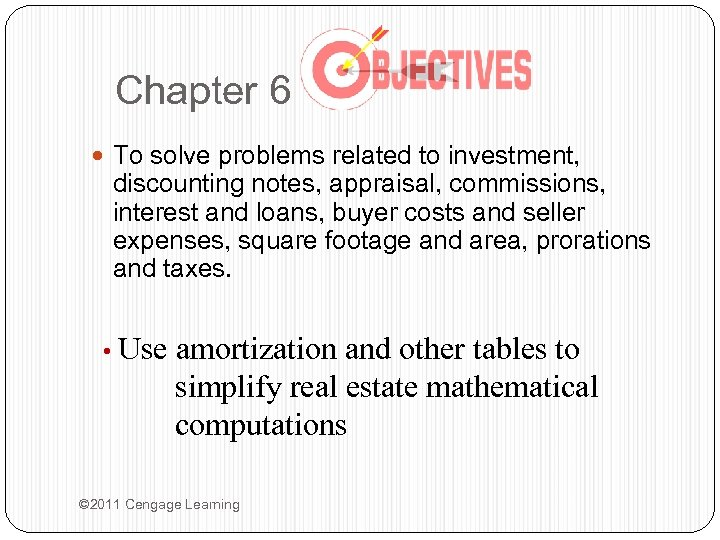Chapter 6 To solve problems related to investment, discounting notes, appraisal, commissions, interest and loans, buyer costs and seller expenses, square footage and area, prorations and taxes. • Use amortization and other tables to simplify real estate mathematical computations © 2011 Cengage Learning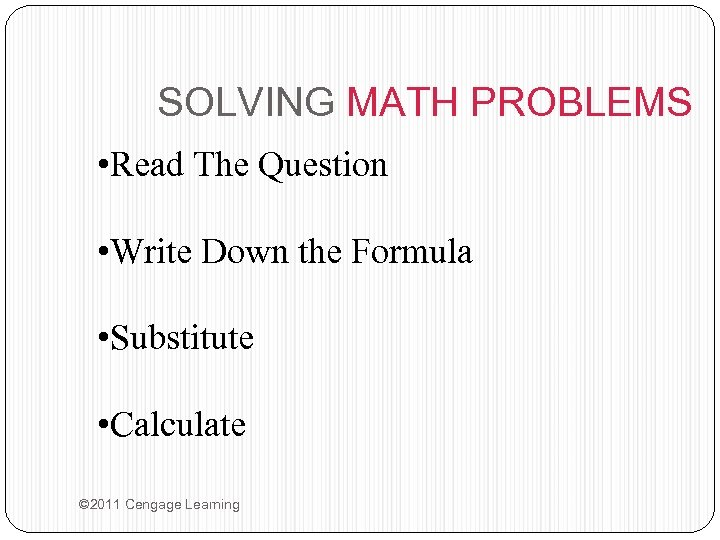SOLVING MATH PROBLEMS • Read The Question • Write Down the Formula • Substitute • Calculate © 2011 Cengage Learning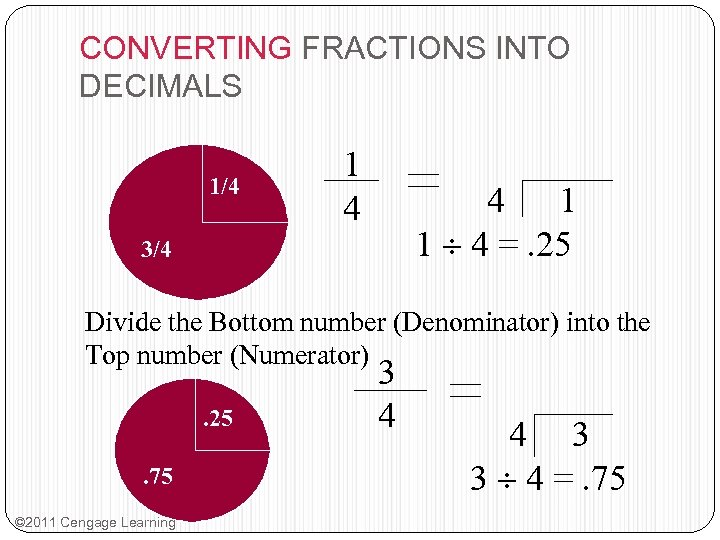CONVERTING FRACTIONS INTO DECIMALS 1/4 1 4 4 1 1 4 =. 25 3/4 Divide the Bottom number (Denominator) into the Top number (Numerator). 25. 75 © 2011 Cengage Learning 3 4 4 3 3 4 =. 75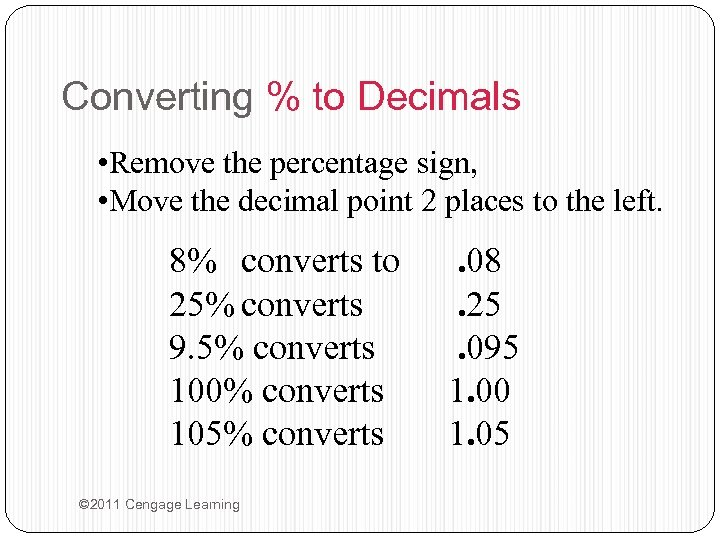Converting % to Decimals • Remove the percentage sign, • Move the decimal point 2 places to the left. 8% converts to 25% converts 9. 5% converts 100% converts 105% converts © 2011 Cengage Learning . 08. 25. 095 1. 00 1. 05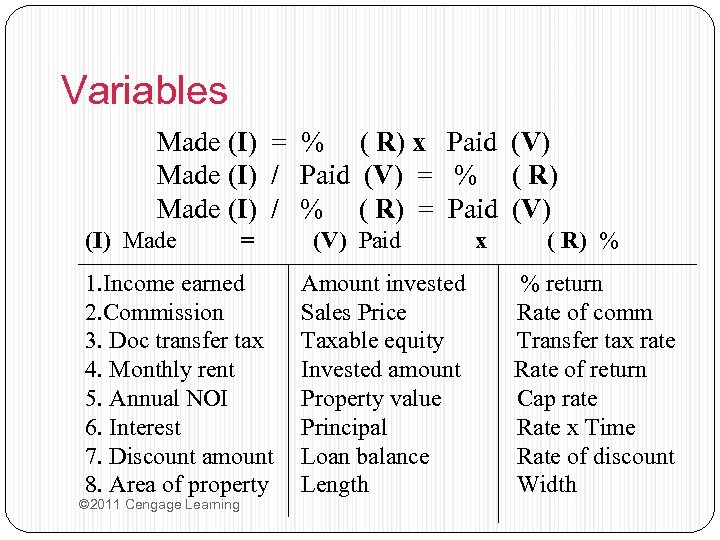Variables Made (I) = % ( R) x Paid (V) Made (I) / Paid (V) = % ( R) Made (I) / % ( R) = Paid (V) (I) Made = 1. Income earned 2. Commission 3. Doc transfer tax 4. Monthly rent 5. Annual NOI 6. Interest 7. Discount amount 8. Area of property © 2011 Cengage Learning (V) Paid Amount invested Sales Price Taxable equity Invested amount Property value Principal Loan balance Length x ( R) % % return Rate of comm Transfer tax rate Rate of return Cap rate Rate x Time Rate of discount Width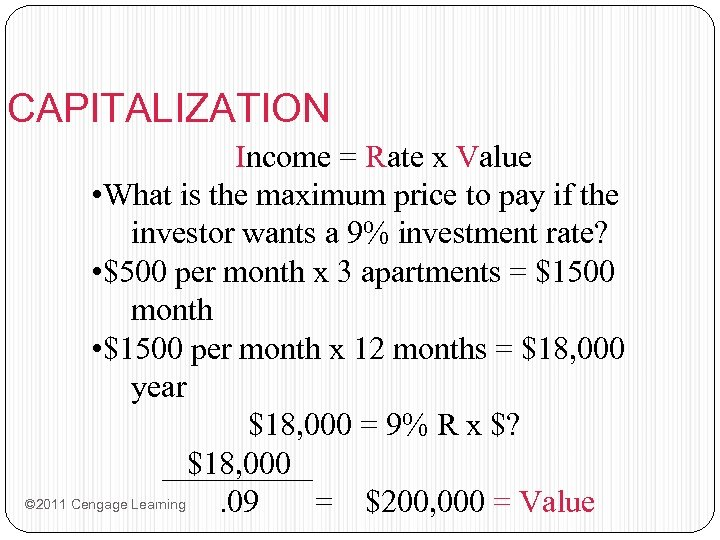CAPITALIZATION Income = Rate x Value • What is the maximum price to pay if the investor wants a 9% investment rate? • \$500 per month x 3 apartments = \$1500 month • \$1500 per month x 12 months = \$18, 000 year \$18, 000 = 9% R x \$? \$18, 000 © 2011 Cengage Learning. 09 = \$200, 000 = Value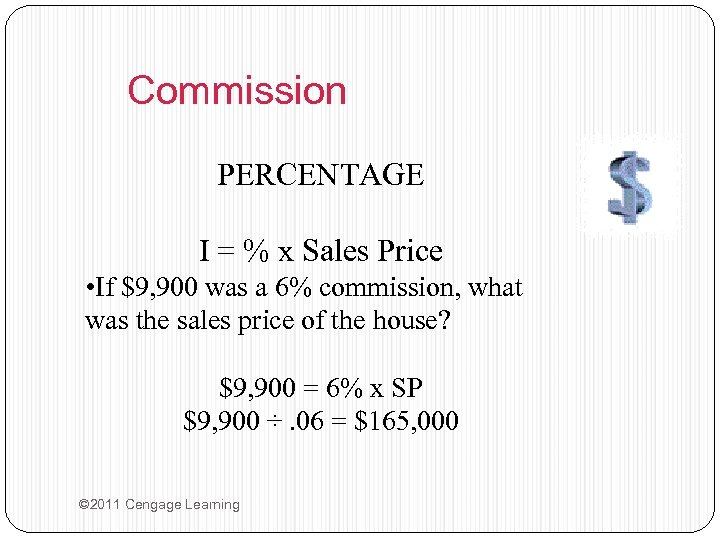Commission PERCENTAGE I = % x Sales Price • If \$9, 900 was a 6% commission, what was the sales price of the house? \$9, 900 = 6% x SP \$9, 900 ÷. 06 = \$165, 000 © 2011 Cengage Learning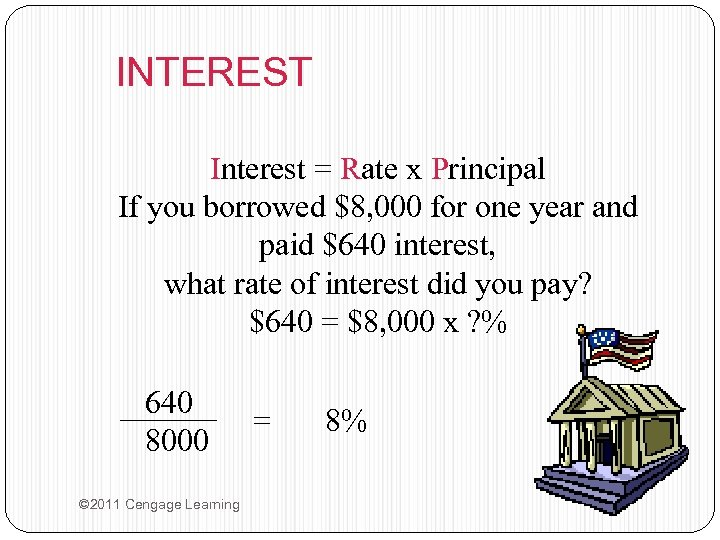INTEREST Interest = Rate x Principal If you borrowed \$8, 000 for one year and paid \$640 interest, what rate of interest did you pay? \$640 = \$8, 000 x ? % 640 8000 © 2011 Cengage Learning = 8%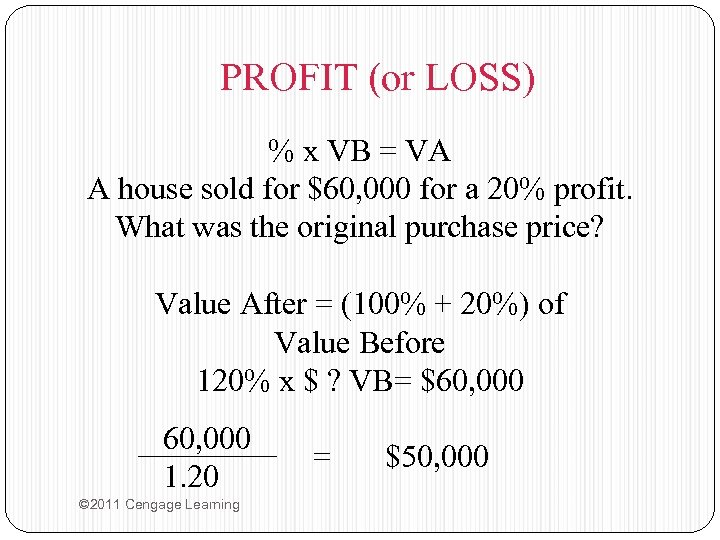PROFIT (or LOSS) % x VB = VA A house sold for \$60, 000 for a 20% profit. What was the original purchase price? Value After = (100% + 20%) of Value Before 120% x \$ ? VB= \$60, 000 1. 20 © 2011 Cengage Learning = \$50, 000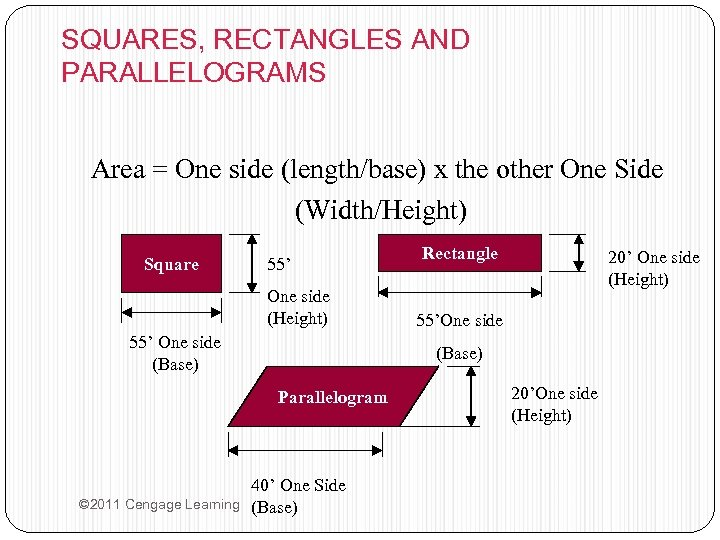SQUARES, RECTANGLES AND PARALLELOGRAMS Area = One side (length/base) x the other One Side (Width/Height) Square 55’ One side (Height) 55’ One side (Base) 20’ One side (Height) 55’One side (Base) Parallelogram © 2011 Cengage Learning Rectangle 40’ One Side (Base) 20’One side (Height)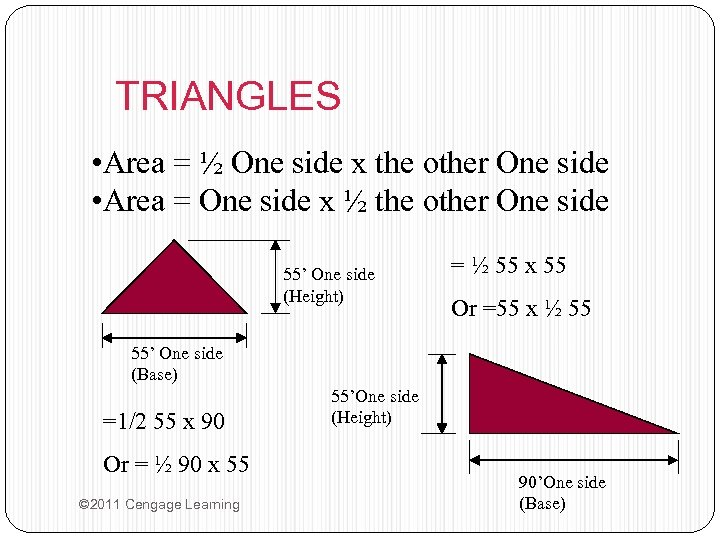TRIANGLES • Area = ½ One side x the other One side • Area = One side x ½ the other One side 55’ One side (Height) = ½ 55 x 55 Or =55 x ½ 55 55’ One side (Base) =1/2 55 x 90 Or = ½ 90 x 55 © 2011 Cengage Learning 55’One side (Height) 90’One side (Base)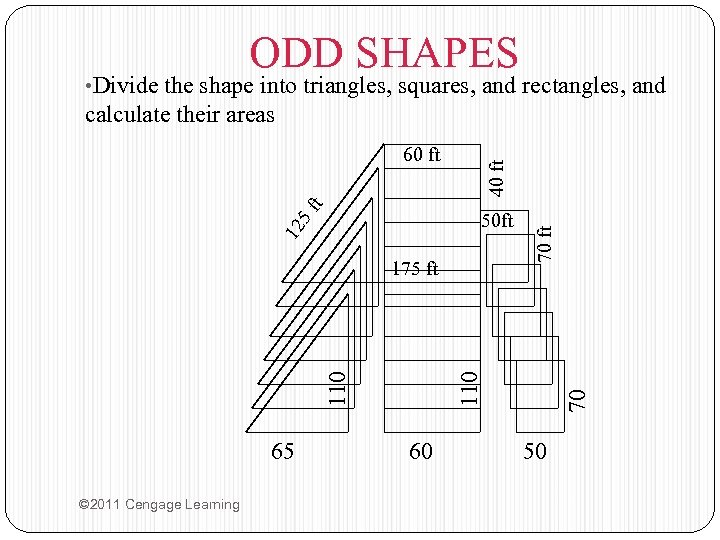ODD SHAPES • Divide the shape into triangles, squares, and rectangles, and calculate their areas 12 40 ft 50 ft 65 © 2011 Cengage Learning 60 70 110 175 ft 70 ft 5 f t 60 ft 50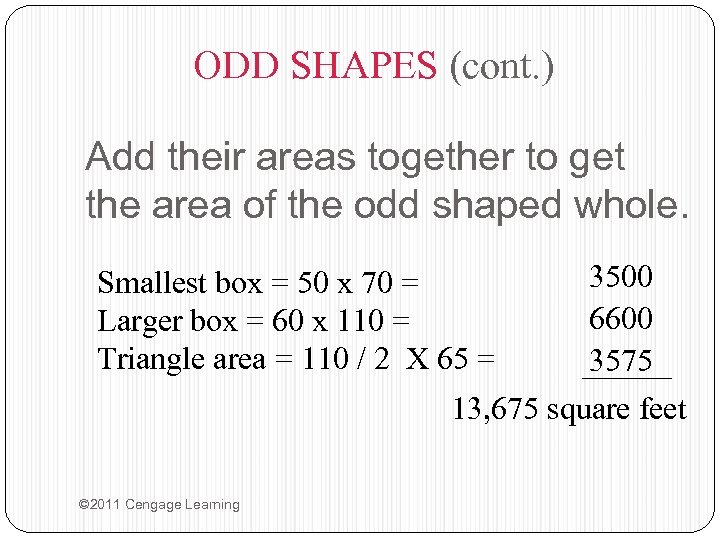ODD SHAPES (cont. ) Add their areas together to get the area of the odd shaped whole. 3500 6600 3575 13, 675 square feet Smallest box = 50 x 70 = Larger box = 60 x 110 = Triangle area = 110 / 2 X 65 = © 2011 Cengage Learning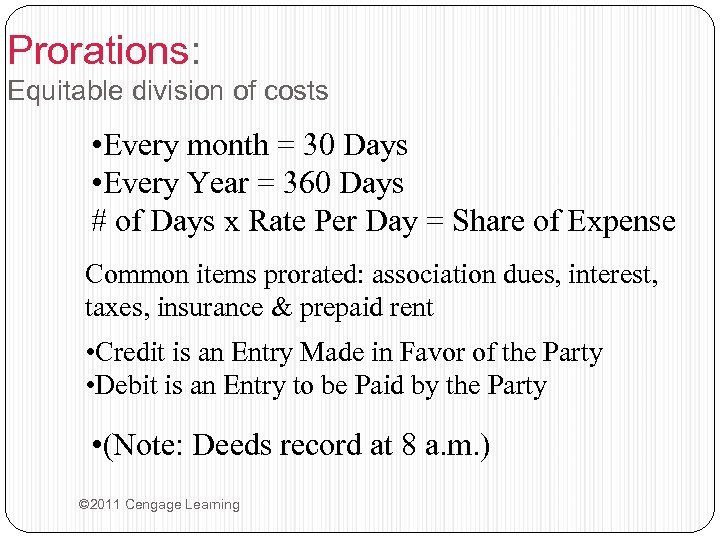Prorations: Equitable division of costs • Every month = 30 Days • Every Year = 360 Days # of Days x Rate Per Day = Share of Expense Common items prorated: association dues, interest, taxes, insurance & prepaid rent • Credit is an Entry Made in Favor of the Party • Debit is an Entry to be Paid by the Party • (Note: Deeds record at 8 a. m. ) © 2011 Cengage Learning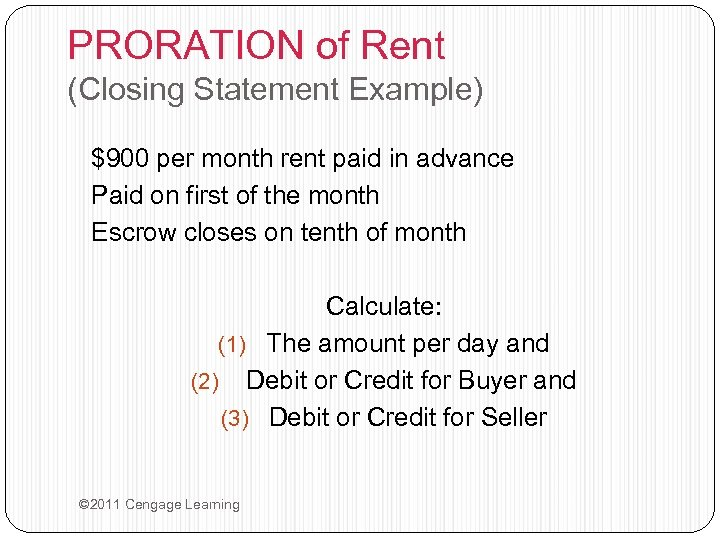PRORATION of Rent (Closing Statement Example) \$900 per month rent paid in advance Paid on first of the month Escrow closes on tenth of month Calculate: (1) The amount per day and (2) Debit or Credit for Buyer and (3) Debit or Credit for Seller © 2011 Cengage Learning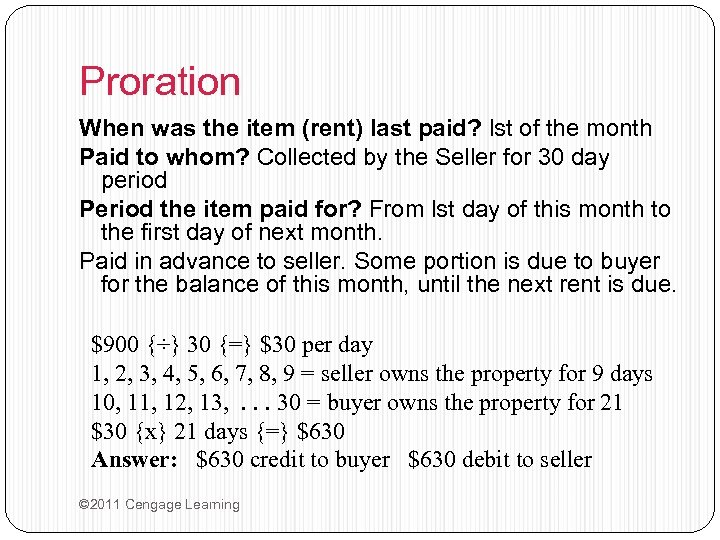Proration When was the item (rent) last paid? lst of the month Paid to whom? Collected by the Seller for 30 day period Period the item paid for? From lst day of this month to the first day of next month. Paid in advance to seller. Some portion is due to buyer for the balance of this month, until the next rent is due. \$900 {÷} 30 {=} \$30 per day 1, 2, 3, 4, 5, 6, 7, 8, 9 = seller owns the property for 9 days 10, 11, 12, 13, . . . 30 = buyer owns the property for 21 \$30 {x} 21 days {=} \$630 Answer: \$630 credit to buyer \$630 debit to seller © 2011 Cengage Learning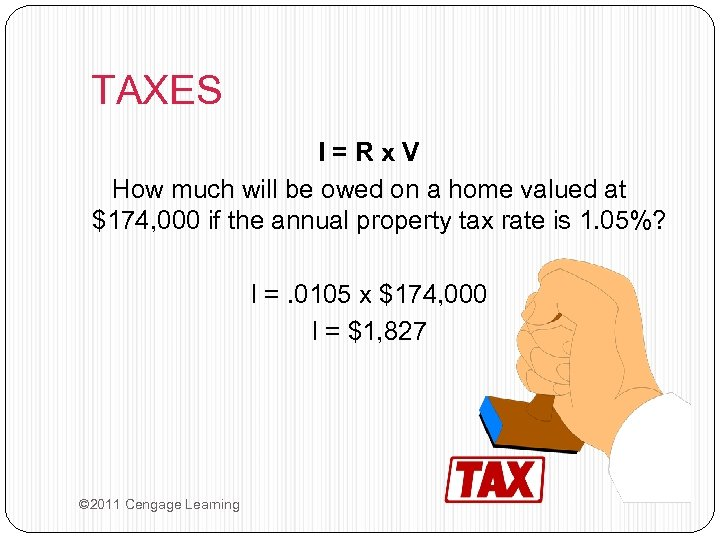TAXES I=Rx. V How much will be owed on a home valued at \$174, 000 if the annual property tax rate is 1. 05%? I =. 0105 x \$174, 000 I = \$1, 827 © 2011 Cengage Learning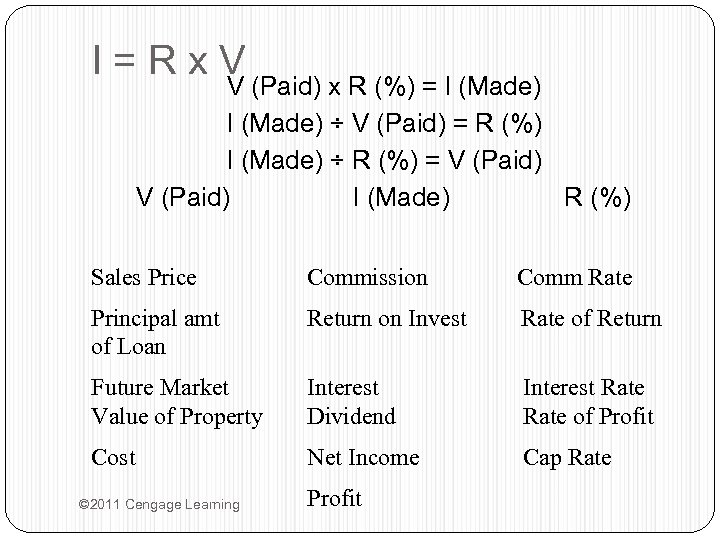I=Rx. V V (Paid) x R (%) = I (Made) ÷ V (Paid) = R (%) I (Made) ÷ R (%) = V (Paid) I (Made) R (%) Sales Price Commission Comm Rate Principal amt of Loan Return on Invest Rate of Return Future Market Value of Property Interest Dividend Interest Rate of Profit Cost Net Income Cap Rate © 2011 Cengage Learning Profit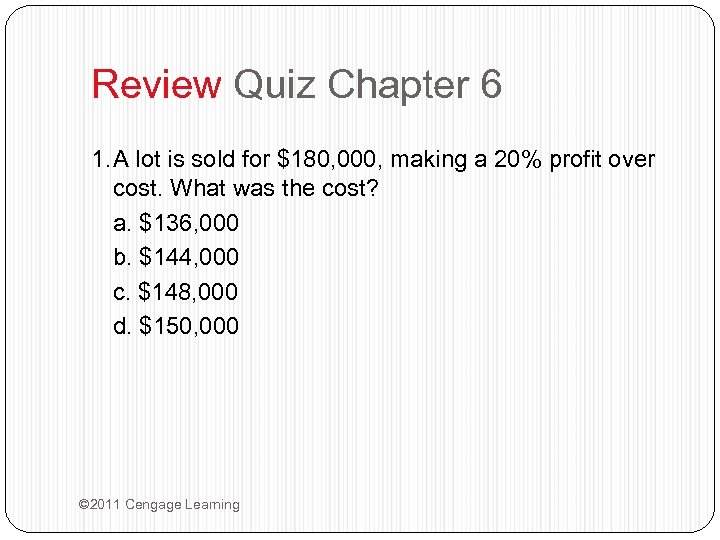Review Quiz Chapter 6 1. A lot is sold for \$180, 000, making a 20% profit over cost. What was the cost? a. \$136, 000 b. \$144, 000 c. \$148, 000 d. \$150, 000 © 2011 Cengage Learning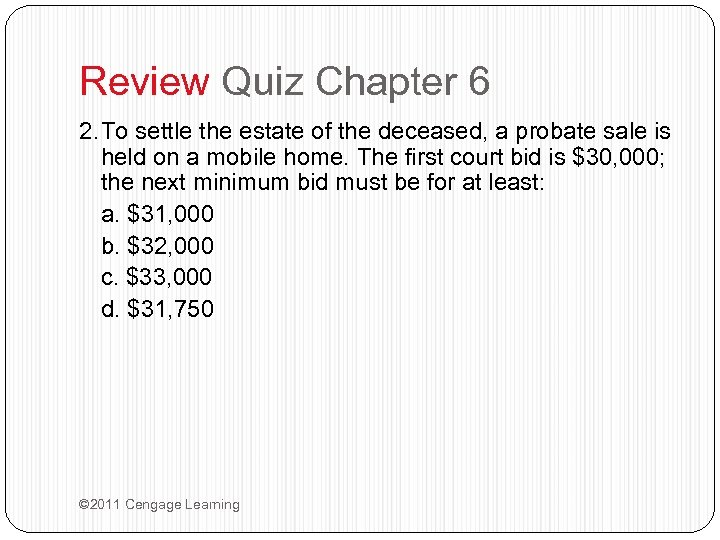Review Quiz Chapter 6 2. To settle the estate of the deceased, a probate sale is held on a mobile home. The first court bid is \$30, 000; the next minimum bid must be for at least: a. \$31, 000 b. \$32, 000 c. \$33, 000 d. \$31, 750 © 2011 Cengage Learning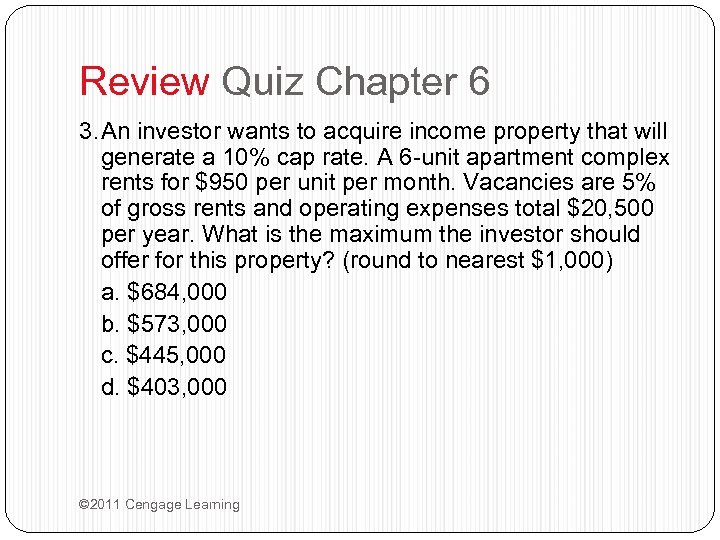Review Quiz Chapter 6 3. An investor wants to acquire income property that will generate a 10% cap rate. A 6 -unit apartment complex rents for \$950 per unit per month. Vacancies are 5% of gross rents and operating expenses total \$20, 500 per year. What is the maximum the investor should offer for this property? (round to nearest \$1, 000) a. \$684, 000 b. \$573, 000 c. \$445, 000 d. \$403, 000 © 2011 Cengage Learning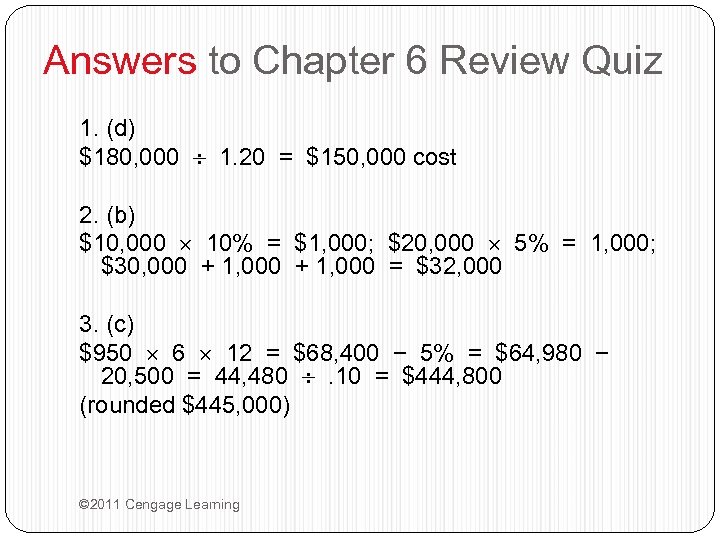Answers to Chapter 6 Review Quiz 1. (d) \$180, 000 1. 20 = \$150, 000 cost 2. (b) \$10, 000 10% = \$1, 000; \$20, 000 5% = 1, 000; \$30, 000 + 1, 000 = \$32, 000 3. (c) \$950 6 12 = \$68, 400 − 5% = \$64, 980 − 20, 500 = 44, 480 . 10 = \$444, 800 (rounded \$445, 000) © 2011 Cengage Learning